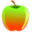CBEST Test Prep

# CBEST Math Test Tips - Page one.

Teaching yourself math concepts can be difficult. The best way is one-on-one tutoring.

Use the whole test booklet as scratch paper! Don't try and cram your calculations in the margin for each problem. You will end up writing smaller and sloppier. Use a good sized blank space not mixed up with anything else. This will help you retrace your steps if you make a mistake.

Be realistic. Just like real estate. The three most important aspects of the math section is Be Realistic, Be Realistic, Be Realistic. If math is your downfall then GET HELP ! You cannot wing this section. The following tips may help improve a score ONLY if you need a little help to get you the extra push over the top. However, eventually you WILL be there so read on!

Know your basic facts. You should be able to readily add, subtract, multiply, and divide small to large numbers IN YOUR HEAD. If you need to use long division to divide 72 by 6, you are in TROUBLE.

Hone your logic skills. Many math questions can actually be answered using only logic and eliminating answers that just don't make sense.

Know some general percentages and what they equal.

1/4 = one fourth = 25% = .25

1/2 = one half = 50% =.50

(Half of ANYTHING is 50%)

3/4 = three fourths = 75% = .75

1/5 = one fifth = 20% = .20

(Any number of fifths is a multiple of 20, i.e.. 2/5 = 40%, 3/5 = 60%, etc.)

Practice finding estimates of percentages of small to large numbers.

Example: 28% of 350. Think: 28% is a little more than 25% or 1/4th. 350 is close to 360 and that is divisible by 4 to get 90. The answer will be around 90. (Correct answer is 98) Try this technique with  a variety of numbers.

Try converting fractions to rounded percentages.

Examples: 545/1100 is about half or 50%. But you should know it is a little below 50%. This will allow you to eliminate all answers 50% or less.

1700/5000 is about 2/5 or 20%. But we know it is a little below 20%. Eliminate answers that are at 20% or higher.

Suppose you have 6/17. You should know that 6/20 is 30%. However, 5/17 is a little MORE than 30%. Eliminate all answers 30% or less.

No calculator is needed (or allowed.) All problems CAN be done in a very short time the old fashioned way.

Sometimes it is easier to pick a wrong answer than a right one. This helps eliminate answers when you are guessing.

More on deciding on wrong answers: Suppose you get to a problem you just cannot do. Don't panic. Save it for last. When you finally get to it, write down all important numbers. Chances are, wrong answers will incorporate something done in a wrong way to these numbers. Think about what you know WILL NOT be done to the numbers. Scan over the answers to see if any of them are a combination of a wrong operation. The following is an example:

Example: There are 100 parking spaces in the faculty parking lot. 2/5 of the spaces are only for compact cars. On Friday, 18 compact cars were parked in the lot. Altogether, the parking lot was 3/4 full. How many cars were not compact cars?

Note: The important numbers are 100, 18, and 3/4. (2/5 is not important. It has nothing to do with the problem, neither does the fact that it is on Friday.) Now then, there is only so much one can do with 100, 18, and 3/4. The answer choices may be as follows:

a. 82

b. 75

c. 118

d. 57

e. 40

To get 82, we would do 100 - 18. Does it make sense to do so? No!

To get 75, that is 3/4 of 100. Does that make sense? Yes, but that just tells how many total cars there are.

To get 118, we add 100 + 18. Does this make sense? No!

To get 57, we find 3/4 of 100 and then subtract 18. Makes sense! BUT, even if you did not realize how to get 57, you would simply not eliminate it. Mark it as a "maybe" answer.

To get 40, we would find 2/5 of 100. Does that make sense? No. Even if you did not realize that 2/5 is not part of the problem, you still should realize 2/5 of 100 is NOT the way to get the answer.

You now have almost been able to eliminate all answers except d. In other words, you are sure the others are wrong, just not so sure if d is the answer. But if you have eliminated all others as indeed being wrong, then it MUST be the answer.

Okay, suppose you could only narrow it down to a choice between d and e.You could guess and have a 50-50 chance now of getting the right answer. Or, you could analyze each answer. You now at least have a starting point to play with. 18 compact cars is a concrete fact. Adding the others to it would give you the total. 57 + 18 = 75, and 40 + 18 = 58. Which makes more sense? Now you can indeed pick answer d! Again, many times the wrong answers listed are just a combination of the important numbers in the problem.

## More CBEST Test Prep Resources

CBEST Test Tips - Overall tips to take and pass the CBEST Test.

CBEST Math Tips - Read our tips to pass the math section of the CBEST Test.

Essay Writing Tips for the CBEST - Get our CBEST essay writing guide here.